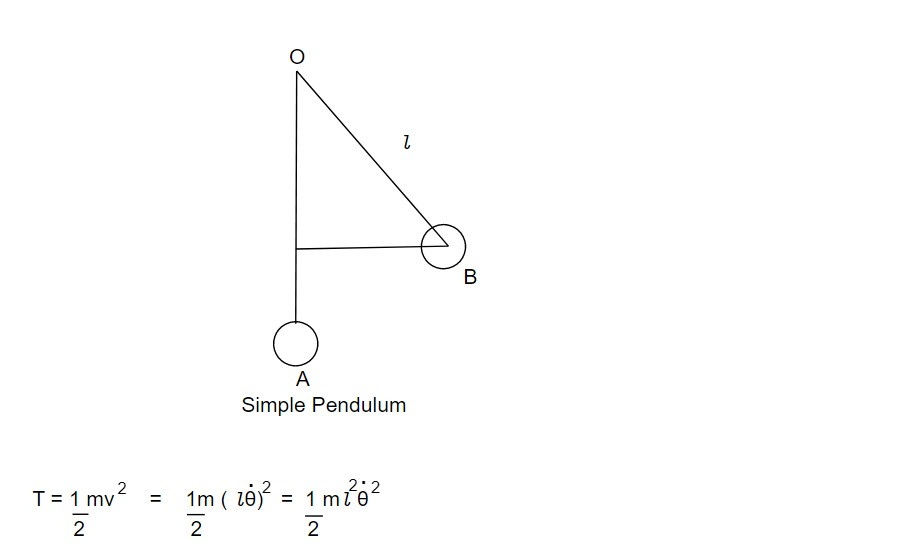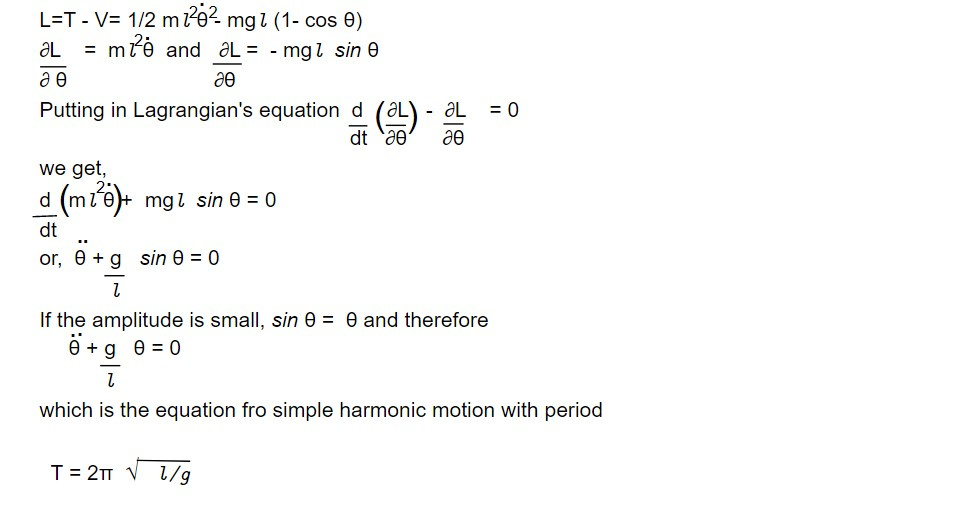# Lagrangian for a simple pendulum and equation describing its motion

The angle θ between rest position and deflected position is chosen as generalized coordinate. If the string is of length l, then kinetic energy iswhere m is the mass of the bob. In moving from position B to A, the mass has fallen freely

through a vertical distance CA. Thus P.E is

V = mg (OA- OC) = mgl (1- cos θ)

where the reference level or zero level of P.E has been taken at a distance l below the point of suspension.This note is taken from Classical Mechanics, MSC, Nepal.